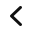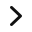# Teaching Division? How to successfully teach it in Third Grade!

Use some of the same strategies to teach multiplication for teaching division in 3rd grade.

Teaching division in 3rd grade can result in the case of the guzintas. What do you mean, you ask? You remember…4 guzinta 36 or 5 guzinta 25. How many of us use to say it out loud as we learned how to divide?

Instead, get rid of the guzintas by teaching division to 3rd graders as how many groups of 4 are in 36? What else matters with how to teach division to kids? For starters, teaching a solid conceptual understanding of division. Using and solving real-life division problems which relate to students. And curing the guzintas!

We also need to be careful we don’t omit a certain type of division problem. What do I mean? When third-graders learn to divide whole numbers we emphasize the quotient will be less than the dividend. But what happens when we divide a whole number by a fraction (6 ÷ ½)? We get a result greater than the dividend! Teaching division using measurement situations will build a solid foundation for later division of fractions in grade 5 and beyond. I will explain more about measurement division later.

Teaching division to 3rd graders has many complexities. In this post, you’ll learn critical strategies for teaching division (both partitive and quotative) to kids conceptually while building procedural fluency. No more guzintas or wondering what exactly happens in a division problem.

## Teaching Division as Fair Share or Equal Groups

One of the first strategies teachers use to teach multiplication is equal groups. Teachers can also use the same strategy for teaching division to kids. This type of division is referred to as partitive division.

While multiplication is an efficient algorithm for finding a total quantity from joining equal groups, division inversely finds the number of groups or the amount in each group. Understanding the context of a story helps students imagine real-life scenarios of how this occurs.

For example, most kids like to bake. If you baked 24 cookies, how many friends could you give cookies to if you gave each friend 8 cookies? or 6 cookies? How about 4 cookies? or 12 cookies? Using dividends (the starting number or total quantity) with multiple divisors (the number that divides) allows students to explore how division works. After all, we want to be fair and share the cookies equally!

## Scenario for Teaching Division to 3rd Graders

Start with presenting scenarios such as the one above to students. Use the CRA (concrete-representational-abstract) approach for teaching division.

• Have students work in pairs to find solutions to how many cookies the friends would get if there were 2, 3, 4, 6, 8, or 12 friends. A wonderful companion book showing this process in story When the Doorbell Rang by Pat Hutchins (I have no affiliate links or association with Amazon).
• Give students math tools such as tiles, counters, beans, or any other object which can be easily grouped.
• Have students make visual representations of their solutions. In other words, go from concrete to represenational.
• Finally, teach the abstract by showing students how to model their solutions with math
• 24 ÷ 3 = 8; 24 ÷ 6 = 4; etc.
• introduce the academic vocabulary of dividend, divisor, quotient and the symbol for division.

## Making the Connection to Multiplication with Arrays

Students must find the connection to multiplication when learning to divide. Some students might immediately connect when they start making equal groups to divide cookies. But some may not, so we need to make the connection explicit for those students.

Since students used arrays to multiply, we can teach division in 3rd grade the same way. In 2nd grade, students lined up objects in rows to use repeated addition to find the total. We can do the same with an array by having students start with a total, then use repeated subtraction to see how many rows (or columns) we can make. This is also a form of partitive division.

## The Same Scenario but Using Arrays

• Have students work in pairs to find solutions to divide 36 brownies among 2, 3, 4, 6 and 9 friends.
• Students should use math tools (tiles, paper squares, beans, counters, etc.) to make rows depending on the divisor (2, 3, 4, 6 or 9).
• Emphasize to students they are to construct an array so rows and columns should be lined up properly and evenly.
• As the students construct the rows or columns, they are conceptually subtracting from the total such as taking 4 more away from the total to make another row/column.
• Provide sentence frames such as 36 brownies arranged in rows of _____ makes _____ rows (36 brownies arranged in rows of 4 makes 9 rows).
• Once the array has been built, have students make a visual respresentation of their solutions.
• Then, ask students to model the solution with mathematics by creating a division equation.
• Finally, ask the students to write a multiplication equation matching the array.

Ask students to notice and wonder once the division and multiplication equations are written (correctly). What do they notice? What do they wonder? Guide the discussion to understand and explain how a division problem is a missing factor problem.

• Where do the factors in the multiplication equation show up in the division equation?
• Do the factors in the multiplication equation represent the same quantities in the division equation?
• How is the quotient and product related?
• How can we use multiplication to solve division problems?

Students have been taught division using equal groups and arrays by this point. These strategies build the concept of division by finding each group’s quantity or the number of groups formed. The teaching of division in 3rd grade usually stops here. But there’s one more strategy to consider.

## Teaching Division Using Measurement

One aspect of teaching division in 3rd grade is sometimes we don’t teach division around measurement problems. What’s a measurement problem? What if I had 6 feet of rope I wanted to cut into 2-foot segments. How many pieces could I cut? This type of division is referred to as quotative division.

How does this differ from the standard division problem? In the situation presented, we know:

• the total (length of the rope)
• we know how many in each group (2-foot segments)
• but we’re trying to solve for how many groups (how many segments).

Though this seems straightforward, it will become more challenging once students in later grades divide by fractions. What if we cut the segments in ½ foot increments (6 ÷ ½)? You would end up with 12 pieces which to students seems counterintuitive when dividing.

So we have to give students opportunities to work with measurement division problems so later they have the experience to understand the context of the problem involving fractions. Also, the rope can be thought of as a number line or measuring ruler. Use a number line as a math tool when teaching division to 3rd graders.

## Teaching Division With a Measurement Scenario

Use measurement scenarios such as the one above. Use the CRA (concrete-representational-abstract) approach in which the concrete model can be a ruler or number line.

• Have students work in pairs to find how many segments can be cut from a 12 inch (use 30 cm or 24 cm if using metric) piece of string if you cut segments of 2, 3, 4, or 6 inch segments.
• Give each pair 4 – 5 strings measuring 12 inches each. Give each pair a simple ruler having inches (or centimeters if using metric).
• Have students use the ruler to divide by 2, 3, or 6 inch segments. If your students are not familiar with using rulers (2nd grade Common Core Math Standards specifically state measuring to the nearest half-inch), you might do a separate lesson on using a ruler to the nearest inch.
• I would recommend the students cut the string into the segments (have extra strings on hand for miscalculations when cutting)
• Provide sentence frames such as a ____ inch string can be divided into ____ segments of ____ inches each (a 12 inch string can be divided into 3 segments of 4 inches each).
• Once the strings have been cut into various segment lengths, have students make a visual represenation of their work. This is when you can supply number lines to substitute for the ruler (because they are the same thing!).
• Then, ask students to model the solution with mathematics by creating a division equation.
• Challenge students to use the missing factor approach by writing an appropriate multiplication sentence.

## What Else?

Are there any other considerations we need to know for teaching division to kids? What about resources? Do you have resources to teach division?

I have a FREEBIE I’m offering if you sign up for my newsletter. It contains 6 pictures you can use to launch a division problem based on equal groups, arrays, or measurement. Based on the Notice and Wonder routine, show the image and have students observe the pictures.

• Shells – a collection of 12 fancy seashells
• Dogs and cats – a collection of 18 dogs or cats (and one mouse – not counted)
• Carton of eggs – 18 eggs arranged in rows of 6
• Rows of crayons – 24 crayons arranged in 3 rows
• Unwound rope – a length of unwound rope (you determine length)
• Yellow ribbon – a length of unwoud ribbon (you determine length)

## Teaching Division with a PowerPoint

A recent addition to my store resources includes a new animated PowerPoint for teaching division to 3rd graders (or review for 4th and 5th graders, intervention students, etc.). The PowerPoint is specifically made to teach dividing through equal groups, arrays, and measurement (number lines).

The PowerPoint includes 4 separate lessons which build on each other

• What is Division?
• Teaching Division with Equal Groups
• Division with Arrays
• Use Measurement Situtations to Teach Division as Subtraction

Each lesson has explicit examples through animation. Each lesson includes the following components:

• concept building with explicit examples with animation mathematical questions to elicit discourse and develop reasoning skills.
• printables for each lesson coordinate with selected slides with hands-on activities to go from concrete understanding to connecting to multiplication symbols and numbers.
• Sentence frames to help frame understanding and support English Language Learners
• Each slide also has sounds to enhance the animation and keep students engaged though it can be turned off by turning down the volume.Teaching Division PowerPoint
Teaching Division PowerPoint
Teaching DIvision PowerPoint
Teaching Division PowerPoint
Teaching Division PowerPoint
Teaching Division PowerPoint
Teaching Division PowerPoint
Teaching Division PowerPointI hope the strategies presented here to teach division to kids were helpful and eliminated any case of the guzintas.

What strategies to do have for teaching division? Share them in the comments!